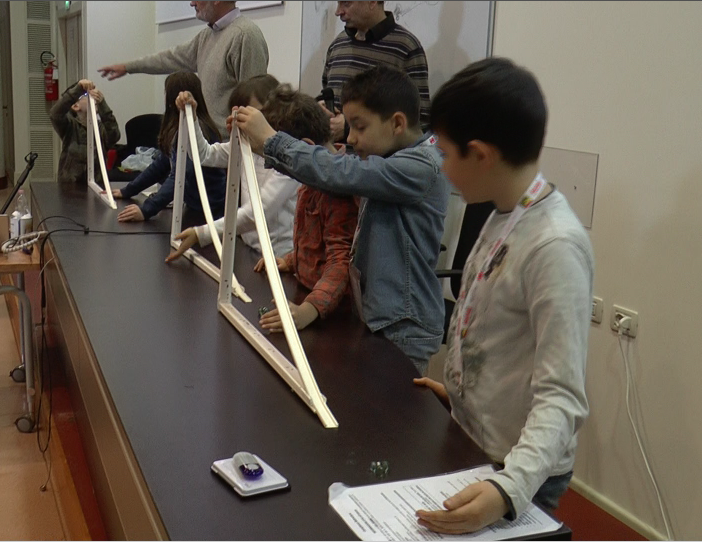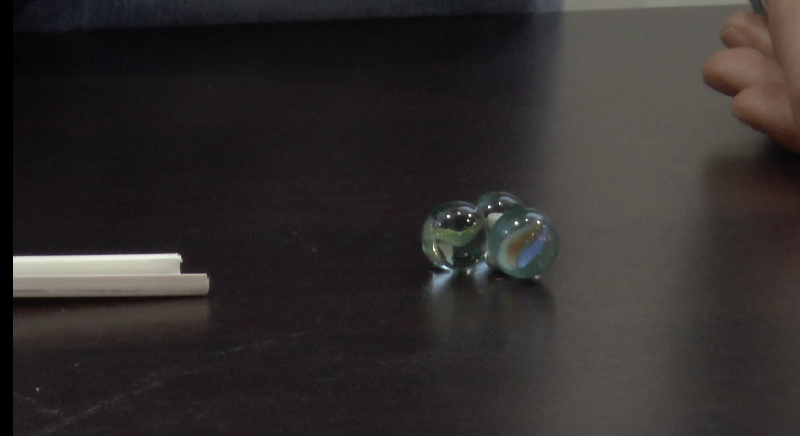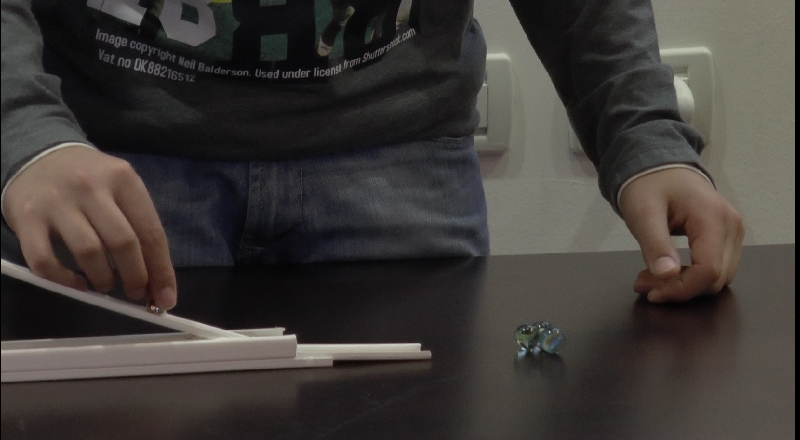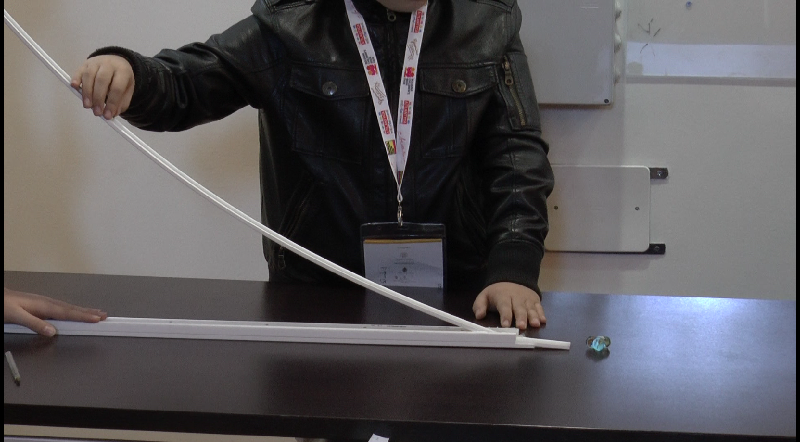Currently there is no translation for this activity.

# Let's Break the Particles

Sandro Bardelli; Amalia PersicoGoals

To have an introduction to potential and kinetic energy, binding energy, the principles of a particle accelerator, and nuclear fission.Learning Objectives
• Demonstrate energy transformation from potential to kinetic, to binding and heat, and again to kinetic energy
• Demonstrate that a marble left to slide from a higher point on the slide has a higher potential energy because it will reach a farther distance.
• Explain the effect friction has on motion by showing the marble coming to a stop.
• There is a threshold energy to break a bound system (like a molecule or an atomic nucleus).
• Scientists use this effect to study matter.Background

Potential energy: Potential energy is the energy “stored” when an object is put in a gravitational field (in our case) at a certain position (in our case height). This “stored” energy can be easily converted to kinetic energy, which is the energy related to the velocity and mass of a body. Furthermore, this kinetic energy is used to break the particles.

Friction: Friction is the resistance to the motion of a body. It is a way to dissipate energy (in our case to change kinetic energy to heat)

Binding energy: Binding energy is the energy required to disassemble a system into separate parts.

Particle collision: In order to see inside atoms, particles and molecules, scientists hit these objects with “bullet particles”. If the kinetic energy of the bullets is more than the binding energy, we open the atoms, particles and molecules and release their constituents.

Particle accelerators: Particle accelerators are devices designed to reach velocities (and therefore the kinetic energy) sufficient to break the particles (see for instance, http://www.cernland.net/).Full Description

This activity is done in three steps.

1) Students release steel marbles at different heights of the slide. They note that if the starting point is higher, the marbles travel further. This indicates that gravity produces kinetic energy.
2) They take glass marbles (usually three) bound by adhesive putty. This system represents a molecule or an atomic nucleus.
3) Starting from the bottom of the slide they reach the minimum height sufficient to separate the marbles when the system is released.

Preparation:

Take plastic tubes and cut it into three pieces of length ~70 cm. Two supporting parts of the main body of the tube are glued with hot glue at an angle of 90 degrees, to make a frame. The final part sits on this structure to make a slide (Figure 1).

(Figure 1)Hypothesis: “Energy cannot be created or destroyed, it can only be changed from one form to another.”
Explanation: There are several forms of energy. Kinetic energy is related to the velocity of a body. But if I brake a car, where does this energy go? Into heat! You can measure the temperature of the brakes after stopping. Simply rub your hands and you will convert kinetic energy into heat.

### Step 1:

Students release steel marbles at different heights on the slide and measure the distance where they stop. The teacher should ask what happened and why. The first observation is that the higher the starting point is on the slide, the further the distance travelled by the marble before it stops.

1) Gravity gives kinetic energy to each marble (more rigorously, transformation from potential to kinetic energy)
2) Friction stops the marble (and the energy is transformed into heat)

Hypothesis: “Objects are bound together and we need energy to break them.” Explanation: What happens when we drop an object (say an egg) from a height of 1 cm or 1 meter? In the first case, some damage is observed on the egg’s surface, while in the second case the egg is destroyed. So, where did the kinetic energy of the egg go? Into breaking the egg. We need a certain amount of energy to break objects, called the “binding energy”.

### Step 2:

Form a clump of glass marbles using a very small amount of adhesive putty (Figure 2). This represents a molecule, an atomic nucleus or more generally a “system”.

(Figure 2)What is the mechanism to break the bond? The bond system needs a certain amount energy to be broken, if the energy is too low, nothing happens.

Keep the glass marble system at the end of the slide and then release the system (figure 3). Progressively increase the height (figure 4) until the energy is enough to separate the balls (see https://www.youtube.com/watch?v=7CHrKoGuZqQ).

(Figure 3)(Figure 4)Try various types of collisions (head-on, off-centred) with more than three marbles. Explain what happens. Why do the marbles separate in some cases?

### Step 3:

In some cases, the binding of the marbles is so strong, that it needs to be released from a higher point, possibly taking too much space in the classroom. Alternatively, another source of energy can be used to break the bound. One possibility is to push the marble with magnets.

What did we learn?
- Energy transforms - Scientists use collisions to explore what the matter is made of.Evaluation
• Make a simple plot relating the height of the starting point of the marble to the distance reached. Ask for a brief explanation of the phenomenon (why different heights of the slide correspond to different effects) and explain the various transformations. Predict the starting height needed for the marble to reach a given distance. What happens if we have a carpet on the floor?
• In some cases, the glass marble system does not break but is shifted. Relate (possibly with a simple graph) the height of the starting point of the steel marble with the shift of the system by varying the number of target glass marbles. The students should realise that the mass of the target also plays a role.
• Students should be able to discuss and give a brief explanation to the following questions:
• Why do different heights give different effects?
• What happens if we use different glues to bind the glass marbles?
• Do we need a longer slide if the glue is super resistant?
• What happens if the collision is not head-on but off-centre?Curriculum
Country Level Subject Exam Board Section
UK KS3 Science - Energy: Energy changes and transfersAdditional Information

For external resources see the following videos: - The story of kinetic and potential energy https://www.youtube.com/watch?v=7K4V0NvUxRg - How does an atom-smashing particle accelerator work? https://www.youtube.com/watch?v=G6mmIzRz_f8 - A quick look around the LHC https://www.youtube.com/watch?v=BEnaEMMAO_sConclusion

The main theme of this activity is how to break particles to study their composition. With a simple device ("the accelerator"), the student studies the effect of collisions between marbles at various heights. This is the time to introduce the important theme of various forms of energy, such as potential, kinetic and binding energy and the possibility of transformation from one form of energy to another.